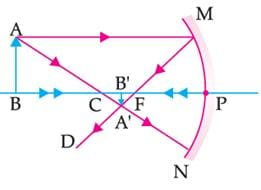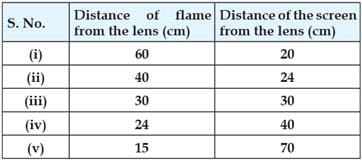# Case Based Questions Test: Light- Reflection & Refraction - 2

## 10 Questions MCQ Test Online MCQ Tests for Class 10 | Case Based Questions Test: Light- Reflection & Refraction - 2

Description
Attempt Case Based Questions Test: Light- Reflection & Refraction - 2 | 10 questions in 20 minutes | Mock test for Class 10 preparation | Free important questions MCQ to study Online MCQ Tests for Class 10 for Class 10 Exam | Download free PDF with solutions
QUESTION: 1

### Following figure illustrates the ray diagram for the formation of images by a concave mirror. The position of the object is beyond the centre of curvature of the concave mirror. On the basis of the given diagram, answer the questions.In case of a concave mirror, the image distance is _________ when image is formed in front of the mirror and _________________ when the image is formed behind the mirror.

Solution: When image is formed in front of the concave mirror, the distance of image is taken as (-) negative and when image is formed behind the mirror, the distance of image is taken as (+) positive.
QUESTION: 2

### Following figure illustrates the ray diagram for the formation of images by a concave mirror. The position of the object is beyond the centre of curvature of the concave mirror. On the basis of the given diagram, answer the questions.A negative sign in the magnification value indicate that the image is ____________

Solution: Negative sign in value of magnification indicates that image is real and inverted because a real image has always negative height i.e. real image is formed below principal axis.
QUESTION: 3

### Read the following passage and answer the questions.A student wants to project the image of a candle flame on the walls of the school laboratory by using a mirror.Which type of mirror should he use and why?

Solution: Concave mirror, it forms real image.
QUESTION: 4

A student wants to project the image of a candle flame on the walls of the school laboratory by using a mirror.

To get the diminished image of the candle flame, the object must be placed at:

Solution: He should place the candle flame between F and 2F (the focus and centre of curvature of the lens) to get the magnified image on the wall while the diminished image is obtained when the object is located at a distance greater than 2F
QUESTION: 5

A student wants to project the image of a candle flame on the walls of the school laboratory by using a mirror. A virtual image formed by concave mirror is:

Solution:

Since we know that virtual image is formed only in case when the object is placed in between focus and pole of concave mirror. The image formed is erect and magnificent (or) enlarged.

QUESTION: 6

A student wants to project the image of a candle flame on a screen 60 cm in front of a mirror by keeping the flame at a distance of 15 cm from its pole.

Find the linear magnification of the image produced.

Solution:

It can be expressed as : Linear Magnification, ⇒ Linear magnification, This shows that the linear magnification produced by a mirror is also equal to the ratio of the image distance (v) to the object distance (u) with a minus sign.

QUESTION: 7

A student wants to project the image of a candle flame on a screen 30 cm in front of a mirror by keeping the flame at a distance of 15 cm from its pole.

What is the distance between the object and its image?

Solution: The distance between the object and its inverted image formed by a concave mirror is 15cm. If the magnification produced by the mirror is -2, use mirror formula to determine the object distance, image distance and focal length of the mirror.
QUESTION: 8

A student focussed the image of a candle flame on a white screen by placing the flame at various distances from a convex lens. He noted his observations as:From the above table, find the focal length of lens without using lens formula:

Solution:

you can see, case (iii); here both the object and the image distances are 30cm .but we know this is possible only case when object kept at 2f, so, 2f = 30cm => f = 15cm
(b) use lens formula for finding incorrect observations.
1/v - 1/u = 1/f
see last observation (v)
u = -15
f = +15
then, 1/v = 1/15 - 1/15 = 0 = 1/∞ => v = ∞
but v = 70cm is given hence, observation (v) is wrong.
(c) obviously case 3 , in this case size of image is equal to size of object due to image distance distance is equal to object distance from pole.

QUESTION: 9

A student focussed the image of a candle flame on a white screen by placing the flame at various distances from a convex lens. He noted his observations as:In which case, the size of the object and image will be same:

Solution:

you can see, case (iii); here both the object and the image distances are 30cm .but we know this is possible only case when object kept at 2f, so, 2f = 30cm => f = 15cm
(b) use lens formula for finding incorrect observations.
1/v - 1/u = 1/f
see last observation (v)
u = -15
f = +15
then, 1/v = 1/15 - 1/15 = 0 = 1/∞ => v = ∞
but v = 70cm is given hence, observation (v) is wrong.
(c) obviously case 3, in this case size of image is equal to size of object due to image distance distance is equal to object distance from pole.

QUESTION: 10

A student focussed the image of a candle flame on a white screen by placing the flame at various distances from a convex lens. He noted his observations as:Which of the following statement is false for the formation of images by convex lens:

Solution:

you can see, case (iii); here both the object and the image distances are 30cm .but we know this is possible only case when object kept at 2f, so, 2f = 30cm => f = 15cm
(b) use lens formula for finding incorrect observations.
1/v - 1/u = 1/f
see last observation (v)
u = -15
f = +15
then, 1/v = 1/15 - 1/15 = 0 = 1/∞ => v = ∞
but v = 70cm is given hence, observation (v) is wrong.
(c) obviously case 3, in this case size of image is equal to size of object due to image distance distance is equal to object distance from pole.Use Code STAYHOME200 and get INR 200 additional OFF Use Coupon Code